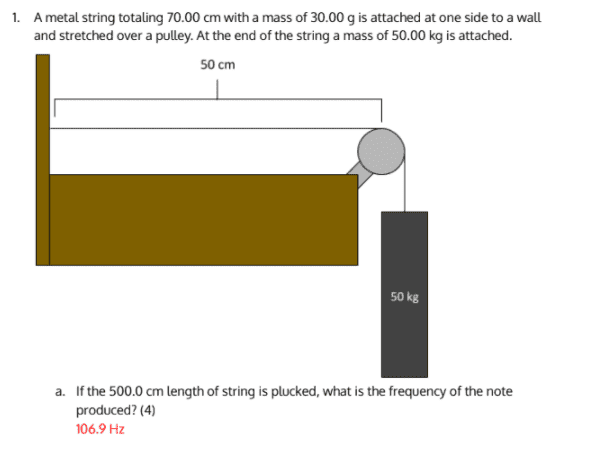# Finding the frequency of a string based on Mass and Tension

SoundsofPhysics
Thread moved from the technical forums to the schoolwork forums
I saw the following problem in a test I was reviewing:I don't understand how they got their answer. I used the formula: ƒ=sqrt(T / u) / 2L where f is the frequency of the string, T is the tension, u is the linear mass density, and L is the length of the string.
I got:
T = mg = 50 * 9.8 = 490N
u = m/l = 3/7 g/cm = 0.04285 kg/m
L = 70cm = 0.7m
Therefore f = sqrt(490 / 0.04285) / 1.4 = 106.93 / 1.4 = 76.38Hz. I see that they got their answer from the first part, but did they forget to divide by 2L, or was I not supposed to do that? Thanks!